## New Maths Curriculum: Year 2 Addition and Subtraction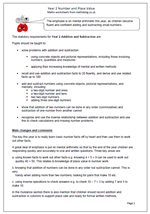Year 2 Addition and subtraction

The emphasis is on mental arithmetic this year, as children become fluent and confident adding and subtracting small numbers.

The statutory requirements for Year 2 Addition and Subtraction are:

Pupils should be taught to:

•    solve problems with addition and subtraction:

using concrete objects and pictorial representations, including those involving numbers, quantities and measures

applying their increasing knowledge of mental and written methods

•    recall and use addition and subtraction facts to 20 fluently, and derive and use related facts up to 100

•    add and subtract numbers using concrete objects, pictorial representations, and mentally, including:
a two-digit number and ones
a two-digit number and tens
two two-digit numbers

•    show that addition of two numbers can be done in any order (commutative) and subtraction of one number from another cannot

•    recognise and use the inverse relationship between addition and subtraction and use this to check calculations and missing number problems.

The key this year is to really learn basic number facts off by heart and then use them to work out other facts.
A great deal of emphasis is put on mental arithmetic so that by the end of the year children are responding quickly and accurately to oral and written questions. Three key areas are:

a. using known facts to work out other facts e.g. knowing 4 + 5 = 9 can be used to work out
quickly 40 + 50. This relates to knowledge of place value in number work.

b. knowing that addition of numbers can be done in any order but subtraction cannot. This is very
handy when adding more than two numbers; looking for pairs that make 10 etc.

c. using inverse operations to check answers e.g. to check 10 – 7 = 3 by adding 7 and 3 to make 10.

In the Guidance section there is also mention that children should record addition and subtraction in columns to support place vale and ready for formal written methods.

New Maths Programme of Study: Year 2 Addition and Subtraction

## New Maths Curriculum: Year 1 Addition and Subtraction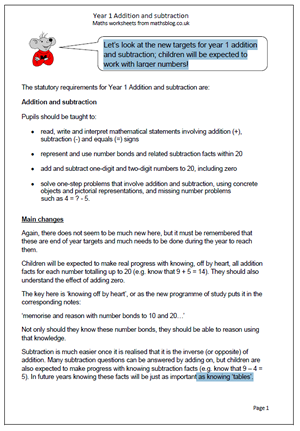Let’s look at the new targets for year 1 addition and subtraction; children will be expected to work with larger numbers!

Pupils should be taught to:

•    read, write and interpret mathematical statements involving addition (+), subtraction (-) and equals (=) signs

•    represent and use number bonds and related subtraction facts within 20

•    add and subtract one-digit and two-digit numbers to 20, including zero

•    solve one-step problems that involve addition and subtraction, using concrete objects and pictorial representations, and missing number problems
such as 4 = ? – 5.

Again, there does not seem to be much new here, but it must be remembered that these are end of year targets and much needs to be done during the year to reach them.
Children will be expected to make real progress with knowing, off by heart, all addition facts for each number totalling up to 20 (e.g. know that 9 + 5 = 14). They should also understand the effect of adding zero.
The key here is ‘knowing off by heart’, or as the new programme of study puts it in the corresponding notes:
‘memorise and reason with number bonds to 10 and 20…’
Not only should they know these number bonds, they should be able to reason using that knowledge.
Subtraction is much easier once it is realised that it is the inverse (or opposite) of addition. Many subtraction questions can be answered by adding on, but children are also expected to make progress with knowing subtraction facts (e.g. know that 9 – 4 = 5). In future years knowing these facts will be just as important as knowing ‘tables’.

New programme of Study: Year 1 Addition and Subtractionddition and subtraction

## Resource of the week: year 1 investigate addition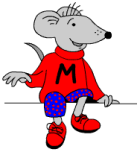Welcome to a new term and plenty of new maths material coming up over the next few months.

From our current resources here is a nice little investigation for young children which will show how well they can organise their thinking and work in a logical way.

The question is simple: how many different ways can you score 8 when throwing two dice?

It’s always a good idea to sit down with your children when doing this type of activity. In this way you can ask questions which will help them clarify their thoughts. The sheet is designed to be used as a record of results. At first they might just keep rolling the dice and adding up the totals until a total of 8 is achieved. Other children might dive straight in with some answers eg 4 + 4 makes 8.

A good question to ask at the end is “How do you know that you have got all the possibilities?

Free Y1 maths worksheet: Investigate dice

## Resource of the Week: written addition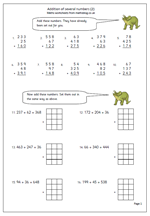Written methods of calculating continue to prove to be some of the more popular pages on the site. This week I would like to highlight a Year 5 maths worksheet on written addition which is the second in a series showing children clearly how addition should be laid out and also giving them the opportunity to re-write questions from a horizontal lay out to a vertical layout. The hardest part of this is to ensure the numbers line up under each other, starting with the units on the far right. Squares can help with this in the early stages, but children should also get used to laying them out correctly without squared paper.

Rarely, if ever, will you find a SATs addition question which has been laid out already in the traditional, or standard method but it is expected that children use this method when solving a difficult addition calculation.

This pager can be found in our Year 5 Calculating section. Similar pages, with more detailed instructions, can also be found in our Four Rules section.

Written addition of several numbers (2)

## More adding three 2-digit numbers mentally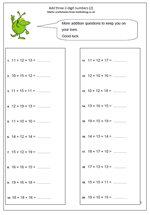I have had a number of requests for more on adding three 2-digit numbers mentally, so, here is another page. There are a number of different techniques which can be used when adding these and it certainly would not be the best way to proceed to just add them in the order they appear. Looking for pairs that make 30, doubling of teens, adjusting by 1 or 2 are just some of the skills needed to answer these quickly and accurately.

Also, don’t forget that correct answers are better than fast incorrect answers, so it is always a good idea to check by adding them in a different order.

Add 3 2-digit numbers practice (2)

This page can be found in the year 5 Calculating category, but many more addition pages can also be found in the four rules section.

## Year 3 maths: adding with 2013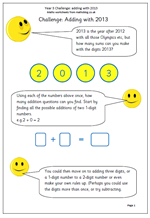Knowing how much I like anything mathematical to do with dates, those kind people at urbrainy.com have let me publish a nifty little investigation to introduce the new year of 2013.

It is a simple idea; how many different addition questions can you find by just using the four digits, 2013.

At first it would be a good idea to look at adding two single digit numbers. Then there are the possibilities of adding a 2-digit number to a single digit; then adding two 2-digit numbers, and so on.

This is good practice at addition as well as encouraging logical thinking and presenting results in a well ordered, methodical way.

There are plenty of extension ideas with this investigation. For example the digits could be used twice, or the numbers could be multiplied rather than added, or even a mixture of the two

e.g. 2 x 0 + 1 = or 2 x 1 + 0 =

Subtraction could also be used, which might well lead on to negative numbers.

This page can be found in the year 3, Using and Understanding Maths category.

## Resource of the Week: year 4 multiplication and addition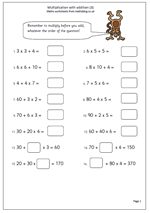This week we are highlighting an important part of year 4 maths which looks at the order of operations.

The rule is that multiplication should be worked out before the addition and in most of these questions that is fairly straightforward. However the questions towards the end are quite tricky.

Let’s look at 70 + ?? x 4 = 150

The best way to go about this is to go in reverse order; subtract 70 from 150 which leaves 80.

Then work out what number multiplied by 4 makes 80, which is 20.

Therefore the missing number is 20.

It is best to check this by going back to the original question; multiply 20 by 4 = 80 and then add 80 and 70 = 150, making the statement true.

Many children will find these very difficult to work out as they require several steps.

## Written method for adding 4-digit numbers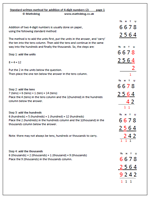Addition of two 4-digit numbers is usually done on paper,using the following standard method:

The method is to add the units first, put the units in the answer, and ‘carry’ the ten into the tens column. Then add the tens and continue in the same way into the hundreds and finally the thousands. So, looking at the addition of 6678 + 2564,

the steps are:

8 + 4 = 12

Put the 2 in the units below the question.
Then place the one ten below the answer in the tens column.
7 (tens) + 6 (tens) + 1 (ten) = 14 (tens)
Place the 4 (tens) in the tens column and the 1(hundred) in the hundreds column below the answer.

6 (hundreds) + 5 (hundreds) + 1 (hundred) = 12 (hundreds)
Place the 2 (hundreds) in the hundreds column and the 1(thousand) in the thousands column below the answer.

Note: there may not always be tens, hundreds or thousands to carry.

6 (thousands) + 2 (thousands) + 1 (thousand) = 9 (thousands)
Place the 9 (thousands) in the thousands column.

More clarification is available on the worksheet, together with a set of additions laid out in the correct format.

Standard addition of 4 digit numbers (2)

## Year 3 maths worksheet: adding 3 numbers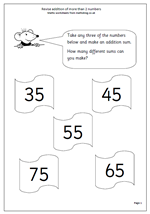Here we have another page of addition which looks at adding three numbers. Each number is a multiple of 5, but there is an extra twist in that children need to work out how many different additions can be made from the numbers available.

A systematic approach is needed, perhaps starting with the largest three numbers;

i.e. 75 + 65 + 55

then moving on to the next largest;

i.e. 75 + 65 + 45

and so on.

An important part of this is to understand that addition can be done in any order so 75 + 65 + 55 is the same as 55 + 65 + 75.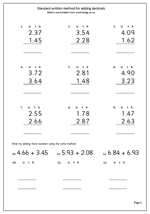Here we have a very straightforward page of addition questions with decimals. All the numbers use 2 decimal places, so it is very similar to adding money. I have also kept to adding just two numbers so this page is ideal for children who are getting to grips with the standard method of addition.